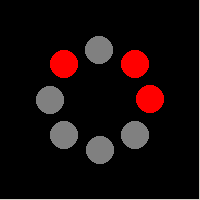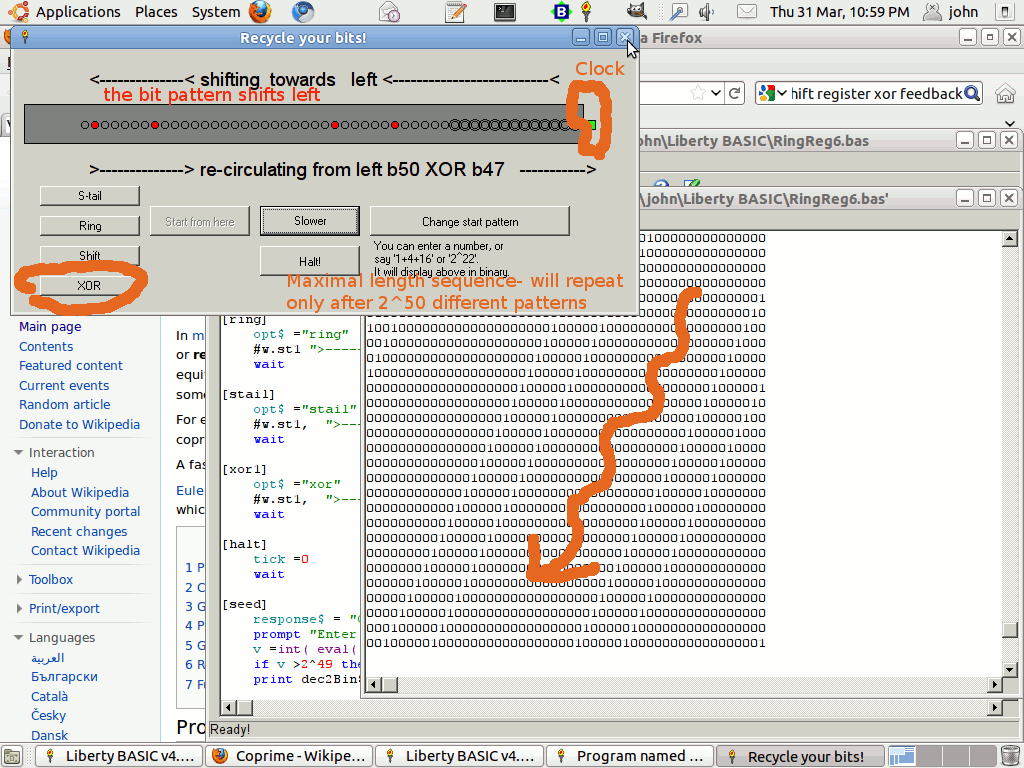# Bit twiddling, Boolean logic, shifting, registers & rotations

Liberty & Just BASIC can do bit and byte twiddling direct, and other operations can be programmed as subroutines, functions, etc.The animations are created programmatically in LB ( see below) via Imagemagick. They show a 8-bit byte being ring-shifted, viewed as a linear display and as a 'clock'. The program allows you to select the pattern you want- choosing '1' ( ie '00000001' is perhaps clearer. '0' or '255' is not very helpful. You also get optical illusion effects- a pattern of ons moving one way is seen as a pattern of offs going the other way- cf. electrons & holes.

## Single bits

A single bit can represent 0 or 1 ( or true/ false). You might want to do Boolean operations between two or more such bits. The operations would be negation, or, and and xor. For instance,

```if power_on and room_cold then call PowerUp
```
Another possibility is toggling an output off/on.
```power =not( power)
```## Bytes- 8 bits at a time

Typical use might be where you are controlling 8 digital output connections. One bit might represent a motor off/on or motor direction. Another might need to pulse briefly high to clock a stepper motor.
For this it is handy to use byte-sized bit twiddling. Since LB does not have built-in input or display in binary, you usually represent a number, for display purposes, via a string variable such as '01010101'. You need dec2binary and binary2decimal routines. I've included in the example a more complex display using drawn filled circles. It helps if you are practiced in 'thinking' in binary and hex as well as decimal. This is common if you have an electronics background.
```outPort =outPort or 4
```
... would be used to force bit two ( of the eight from 0 to 7) high. ( 4 is '00000100' in binary.)
```outPort =outPort and 251
```
... would force the same bit low. ( 251 is '11111011' in binary.)

There are several other useful operations, usually referred to in electronics as 'shift registers'. The bits in the byte can be shifted left or right, losing a bit at one end and feeding in a zero at the other. Alternatively they can be rotated either way- here, the bit 'falling off' one end is fed back in at the other end. You can also feedback in the negated overflow bit.

## Longer registers

While in hardware we usually use either one bit; 8-bit bytes; or multiples of eight bits, it is easy in software to use ANY length. This can be useful for instance in making GUI analog clocks; or to create a rotary encoder.

There is also the interesting possibility of other feedback ring-counters. Especially interesting is the maximal-length switch-tail ring-counter, where XOR-ing an appropriate pair of bits can create a pseudo-random sequence.

## CAUTION!

```Booleans are a bit problematic in LB. There is no boolean type, nor any boolean logic operators in LB.
The IF-statement treats any non-zero value as true.
Comparison operators evaluate to 1 (true) or 0 (false).
Some other functions use -1 for true (such as the not() function).

AND, OR and XOR are bitwise operators.

An example of how you can get bitten if you disregard this and think that you can really use any value for True, and that bitwise operators are really logical operators:

False = 0
True = 2

a = 1
b = 1
if a = b and True then
print "True and True is True"
else
print "AND fails on two true values"
end if

if (a = b) xor True then
print "...and we fail with XOR"
else
print "True xor True is False"
end if

The difference between logical and bitwise AND:
The operands of logical and are boolean values (1 logical_and 2 = true and true = true),
the operands of bitwise and are numeric values (1 bitwise_and 2 = 0).

LB has only the latter kind of AND.
```

A second program allows you to experiment with simple 50-bit shift registers, allowing you to enter arbirary bit-patterns then clock them leftwards. You choose whether to lose the overflow; shift it in at the right; invert it and shift in at the right; or XOR two bits and feed the result back in, to investigate maximal length chain code generation & PRNGs.The code below is an example of using LB in such situations. It includes functions to do the shifts and rotates and to display them. I'll be modifying this page and adding to it.
```
mainwin 80 30

nomainwin

UpperLeftX   =  10
UpperLeftY   =  10
WindowWidth  = 400
WindowHeight = 400

graphicbox #w.gb1, 50, 30, 300, 40
graphicbox #w.gb2,150, 90, 200,200

textbox    #w.tb1, 150,300, 200, 20

button     #w.b1,  "Ring-L",    [ring],   LR, 310, 200, 70, 30
button     #w.b2,  "Seed",      [seed],   LR, 310, 250, 70, 30
button     #w.b3,  "L-shift",   [lshift], LR, 310, 150, 70, 30
button     #w.b4,  "R-shift",   [rshift], LR, 310, 100, 70, 30
button     #w.b5,  "Pattern",   [pattern],LR, 310,  50, 70, 30

open "Bitwise Demos" for window as #w

#w,     "trapclose [quit]"

#w.gb1, "down"
#w.gb1, "fill black ; flush"
#w.gb1  "backcolor darkgray"

for i =1 to 8
#w.gb1 "up ; goto "; 34 *i -5; " 20"
#w.gb1 "down ; circlefilled 15"
next i

#w.gb1 "flush"

#w.gb2, "down"
#w.gb2, "fill black ; flush"
#w.gb2  "backcolor darkgray"

for i =1 to 8
theta =i /8 *2 *3.14159265
xx =50 *cos( theta)
yy =50 *sin( theta)
#w.gb2 "up ; goto "; 100 +xx; " "; 100 +yy
#w.gb2 "down ; circlefilled 15"
next i

#w.gb1 "flush"

v =int( 256 *rnd( 1))
#w.tb1 v; " Edit to any integer 0--255"
print dec2Bin\$( v, 8)

wait

[pattern]
#w.tb1 "!contents? v"
print dec2Bin\$( v, 8)
wait

[ring]
for i =1 to 16
print dec2Bin\$( v, 8)
v =rotLeft( v)
#w.gb1 "getbmp scr 0 0 300 40"
bmpsave "scr", "scr"; i; ".bmp"
'  ImageMagick-  'convert -delay 100 -loop 0 scr*.bmp anim.gif'
timer 1000, [on]
wait
[on]
timer 0
next i

wait

[seed]
v =int( 256 *rnd( 1))
#w.tb1 v
print dec2Bin\$( v, 8)
wait

function dec2Bin\$( num, b)  '   returns a string, length 16
'   leading spaces then the binary rep'n in b bits.
while ( num >0)
dec2Bin\$ =str\$( num mod 2) +dec2Bin\$
num =int( num /2)
wend
'l =len( dec2Bin\$)
dec2Bin\$ =right\$( "00000000" +dec2Bin\$, b)
dec2Bin\$ =right\$( space\$( 16) +dec2Bin\$, 16)
for i =9 to 16
if mid\$( dec2Bin\$, i, 1) ="1" then
#w.gb1 "backcolor red"
#w.gb2 "backcolor red"
else
#w.gb1 "backcolor darkgray"
#w.gb2 "backcolor darkgray"
end if
#w.gb1 "up ; goto "; 34 *( i -8) -5; " 20"
#w.gb1 "down ; circlefilled 15"
theta =( i -8) /8 *2 *3.14159265
xx =50 *cos( theta)
yy =50 *sin( theta)
#w.gb2 "up ; goto "; 100 +xx; " "; 100 +yy
#w.gb2 "down ; circlefilled 15"
next i
end function

[lshift]
for i =1 to 8
print dec2Bin\$( v, 8)
v =shiftLeft( v)
timer 1000, [on2]
wait
[on2]
timer 0
next i

wait

[rshift]
for i =1 to 8
print dec2Bin\$( v, 8)
v =shiftRight( v)
timer 1000, [on3]
wait
[on3]
timer 0
next i

wait

[quit]
close #w

end

function shiftLeft( bitsValue)
shiftLeft =bitsValue *2
end function

function shiftRight( bitsValue)
shiftRight = int( bitsValue / 2)
end function

function shiftByteLeft( bitsValue)
shiftByteLeft = 255 and ( bitsValue *2)
end function

function rotLeft( x)
rotLeft =( (x +x) mod 256) or (x >127)
end function

function rotRight( x)
rotRight =( 128 *( x and 1)) or int( x /2)
end function

function inv\$( x)   '   8-bit bit inversion
j\$ =right\$( dec2Bin\$( x, 8), 8)
k\$ =""
for j =1 to 8
if mid\$( j\$, j, 1) ="1" then l\$ =l\$ +"0" else l\$ =l\$ +"1"
next j
inv\$ =right\$( l\$, 8)
end function
```

Prog 2```
'nomainwin

UpperLeftX   =  10
UpperLeftY   =  10
WindowWidth  = 630
WindowHeight = 290

graphicbox #w.gb1,  10,  55, 560,  40

button     #w.b1, "Faster",                     [speed],      LR, 350, 80, 100, 30
button     #w.b7, "Halt!",                      [halt],       LR, 350, 40, 100, 30

button     #w.b2, "Start from here",            [PatChange],  LR, 460, 80, 100, 30
button     #w.b3, "Change start pattern",       [seed],       LR, 240, 80, 200, 30

button     #w.b6, "S-tail",                     [stail],      LR, 570,100, 100, 20
button     #w.b5, "Ring",                       [ring],       LR, 570, 70, 100, 20
button     #w.b4, "Shift",                      [shift],      LR, 570, 40, 100, 20
button     #w.b8, "XOR",                        [xor1],       LR, 570, 10, 100, 20

statictext #w.st1,  "", 75,110, 510, 24
statictext #w.st2,  "<--------------< shifting  towards   left <--------------------------<", 75, 20, 510, 24
statictext #w.st3, "You can enter a number, or say '1+4+16' or '2^22'."; chr\$( 13);_
"It will display above in binary.", 360, 190, 140, 50

'textbox    #w.tb1, 456, 157, 60, 30

graphicbox #w.gb2, 572,  71,  10,  10

del  =1000
opt\$ ="shift"
tick =1

global v

open "Recycle your bits!" for window as #w

#w,     "trapclose [quit]"

#w.gb1, "down"
#w.gb2  "down ; goto 4 4 ; down ; backcolor red ; color red ; fill black"
#w.gb1  "fill darkgray ; flush"
#w.gb1  "backcolor darkgray"
#w.st1  "!font arial 14"
#w.st2  "!font arial 14"
'#w.tb1  "!font arial 14"

for i =1 to 50
#w.gb1 "up ; goto "; 10 *i +50; " 20"
#w.gb1 "down ; circlefilled 6"
next i

#w.gb1 "flush"

v =   1

'print v
'#w.tb1 v

dummy\$ =dec2Bin\$( v)

wait

[shift]
opt\$ ="shift"
#w.st1 ""
wait

[ring]
opt\$ ="ring"
#w.st1 ">--------------> re-circulating from left >-------------------------->"
wait

[stail]
opt\$ ="stail"
#w.st1,  ">--------------> re-circulating from left but inverted  ----------->"
wait

[xor1]
opt\$ ="xor"
#w.st1,  ">--------------> re-circulating from left b50 XOR b47   ----------->"
wait

[halt]
tick =0
wait

[seed]
response\$ = "C:"
prompt "Enter an integer seed 0 -- 2^49"; v\$
v =int( eval( v\$))
if v >2^49 then v =2^49
print dec2Bin\$( v)

[PatChange]
'#w.b2, "Start"
#w.b2 "!disable"
del =1000
'#w.tb1 v
#w.b1 "Faster"
t =0
tick =1
while tick =1
#w.gb2 "backcolor green ; circlefilled 7"
print dec2Bin\$( v)
#w.gb1 "getbmp scr 0 10 560 20"
'bmpsave "scr", "scr"; right\$( "00000" +str\$( t), 5); ".bmp"
'these will be merged into an animation with ImageMagick
t =t +1
timer del, [on0]
wait
[on0]
timer 0

select case opt\$
case "shift"
v =lshift( v)
case "ring"
v =lrot( v)
case "stail"
v =stail( v)
case "xor"
v =fxor( v)
end select

#w.gb2 "backcolor black ; circlefilled 7"

timer del, [on]
wait
[on]
timer 0
wend
#w.b2 "!enable"
wait

[speed]
if del =1000 then
del =50
#w.b1 "Slower"
else
del =1000
#w.b1 "Faster"
end if

if v =1 then del =1000
wait

function dec2Bin\$( num)
while ( num >0)
dec2Bin\$ =str\$( num mod 2) +dec2Bin\$
num      =int( num /2)
wend

dec2Bin\$ =right\$( "0000000000000000000000000000000000000000000000000000000000000000000000000000000000000000" +dec2Bin\$, 50)

for i =1 to 50
if mid\$( dec2Bin\$, i, 1) ="1" then
#w.gb1 "backcolor red"
else
#w.gb1 "backcolor darkgray"
end if

#w.gb1 "up ; goto "; 10 *i +50; " 20"
#w.gb1 "down ; circlefilled 4"
next i
end function

[quit]
close #w
end

function lrot( x)
lrot =( (x +x) mod 2^50) or (x >=2^49)
end function

function lshift( x)
lshift =( x +x) mod 2^50
end function

function stail( x)
stail =( ( x +x) mod 2^50) or ( (x >=2^49) =0)
end function

function fxor( x)
vv   =x
Bin\$ =""

while ( vv >0)
Bin\$ =str\$( vv mod 2) +Bin\$
vv      =int( vv /2)
wend

Bin\$ =right\$( "0000000000000000000000000000000000000000000000000000" +Bin\$, 50)
'print Bin\$

b1 =val( mid\$( Bin\$, 1, 1))
if b1 <>0 then b1 =1 else b1 =0

b2 =val( mid\$( Bin\$, 4, 1))
if b2 <>0 then b2 =1 else b2 =0

carry =b1 xor b2
if carry <>0 then carry =1 else carry =0

'print b1, b2, carry

fxor =( (x +x) mod 2^50) or carry
end function

```